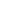# Free Class 4 Area and perimeter of rectangle Worksheets

Download free printable Area and perimeter of rectangle Worksheets to practice. With thousands of questions available, you can generate as many Area and perimeter of rectangle Worksheets as you want.

## Sample Class 4 Area and perimeter of rectangle Worksheet Questions

1.

Determine the L of the following, if Perimeter = 48 cm2.

Determine the L of the following, if Perimeter = 36 m3.

Determine the L of the following, if Perimeter = 24 cm4.

Determine the L of the following, if perimeter = 22 cm5.

Determine the W of the following, if Perimeter = 30 cm.6.

Determine the W of the following, if Perimeter = 18 cm.7.

Determine the W of the following, if Perimeter = 20 cm8.

Determine the W of the following, if Perimeter = 28 m9.

Determine the W of the following, if Perimeter = 22 cm10.

Determine the value of W, if Area = 63 cm sq.Worksheets by UrbanPro

Our worksheets are designed to help students explore various topics, practice skills and enrich their subject knowledge, to improve their academic performance. Designed by Experts who have extensive experience and expertise in teaching a subject, these worksheets will improve your child's problem-solving skills and subject knowledge in a fun and interactive manner.
Check out our free customized worksheets across school boards, grades, subjects and levels of subject knowledge. You can download, print and share these worksheets with anyone, anywhere, anytime!

Get a custom worksheet to practice!

Select your topic & see the magic.

subjectSelect Chapter(s)

Chapters & Subtopics